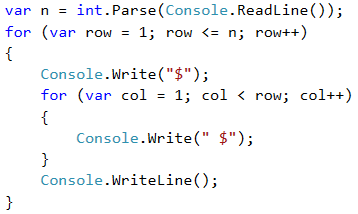# Example: Triangle Made of Dollars

Write a program that takes an integer n and prints a triangle made of dollars of size n.

Input Output Input Output Input Output
3 $ $ $ $ 
4 $ $ $ $ 
 
5 $ $ $ $ 
 
  \$

## Video: Triangle of Dollars

Watch this video to learn how to print a triangle of dollars on the console, using nested for-loops: https://youtu.be/Pbfe1F0nMNE.

## Hints and Guidelines

The problem is similar to those for drawing a rectangle and square. Once again, we will use nested loops, but there is a catch here. The difference is that the number of columns that we need to print depends on the row, on which we are and not on the input number n. From the example input and output data we see that the count of dollars depends on which row we are on at the moment of the printing, i.e. 1 dollar means first row, 3 dollars mean third row and so on. Let's see the following example in detail. We see that the variable of the nested loop is connected with the variable of the outer one. This way our program prints the desired triangle.## Testing in the Judge System

Test your solution here: https://judge.softuni.org/Contests/Practice/Index/512#3.